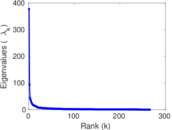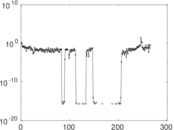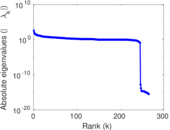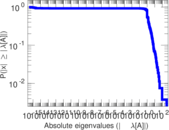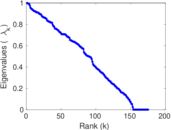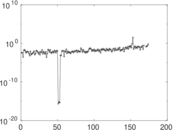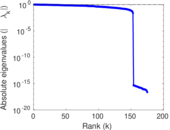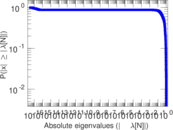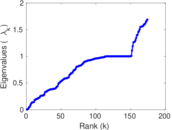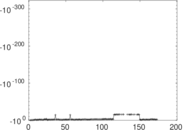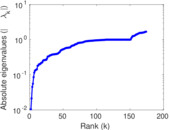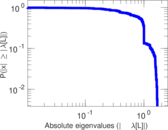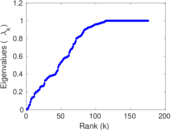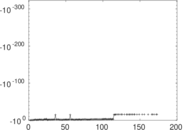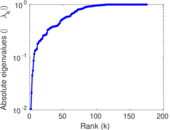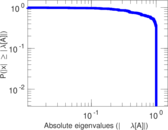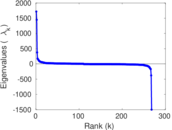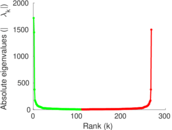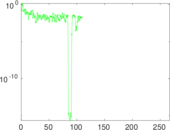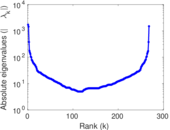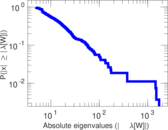# Wikiquote edits (la)

This is the bipartite edit network of the Latin Wikiquote. It contains users and pages from the Latin Wikiquote, connected by edit events. Each edge represents an edit. The dataset includes the timestamp of each edit.

 Code `qla` Internal name `edit-lawikiquote` Name Wikiquote edits (la) Data source http://dumps.wikimedia.org/ AvailabilityDataset is available for download Consistency checkDataset passed all tests Category Authorship network Dataset timestamp 2017-10-20 Node meaning User, article Edge meaning Edit Network formatBipartite, undirected Edge typeUnweighted, multiple edges Temporal dataEdges are annotated with timestamps

## Statistics

 Size n = 1,681 Left size n1 = 268 Right size n2 = 1,413 Volume m = 5,685 Unique edge count m̿ = 2,637 Wedge count s = 166,918 Claw count z = 13,406,892 Cross count x = 999,901,208 Square count q = 35,308 4-Tour count T4 = 956,318 Maximum degree dmax = 1,985 Maximum left degree d1max = 1,985 Maximum right degree d2max = 449 Average degree d = 6.763 83 Average left degree d1 = 21.212 7 Average right degree d2 = 4.023 35 Fill p = 0.006 963 59 Average edge multiplicity m̃ = 2.155 86 Size of LCC N = 1,378 Diameter δ = 13 50-Percentile effective diameter δ0.5 = 3.630 50 90-Percentile effective diameter δ0.9 = 5.897 78 Median distance δM = 4 Mean distance δm = 4.372 28 Gini coefficient G = 0.769 987 Balanced inequality ratio P = 0.187 951 Left balanced inequality ratio P1 = 0.123 659 Right balanced inequality ratio P2 = 0.264 028 Relative edge distribution entropy Her = 0.820 145 Power law exponent γ = 3.300 57 Tail power law exponent γt = 2.181 00 Tail power law exponent with p γ3 = 2.181 00 p-value p = 0.000 00 Left tail power law exponent with p γ3,1 = 1.721 00 Left p-value p1 = 0.410 000 Right tail power law exponent with p γ3,2 = 2.371 00 Right p-value p2 = 0.000 00 Degree assortativity ρ = −0.345 886 Degree assortativity p-value pρ = 5.537 09 × 10−75 Spectral norm α = 377.339 Algebraic connectivity a = 0.010 435 3 Spectral separation |λ1[A] / λ2[A]| = 3.993 62 Controllability C = 1,168 Relative controllability Cr = 0.704 463

## Plots

### Fruchterman–Reingold graph drawing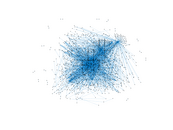### Degree distribution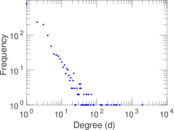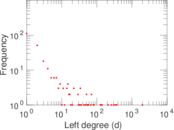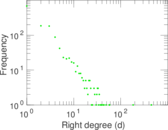### Cumulative degree distribution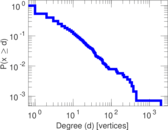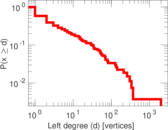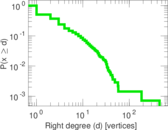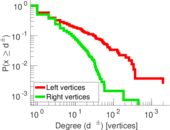### Lorenz curve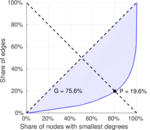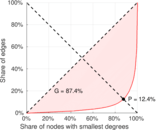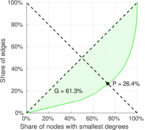### Spectral distribution of the adjacency matrix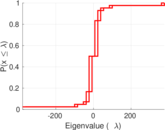### Spectral distribution of the normalized adjacency matrix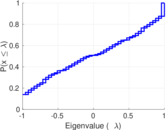### Spectral distribution of the Laplacian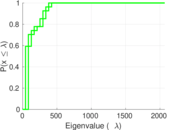### Spectral graph drawing based on the adjacency matrix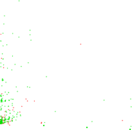### Spectral graph drawing based on the Laplacian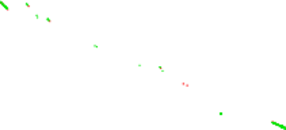### Spectral graph drawing based on the normalized adjacency matrix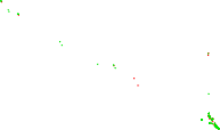### Degree assortativity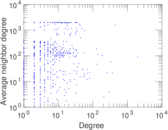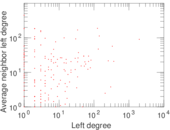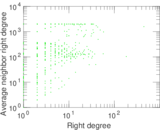### Zipf plot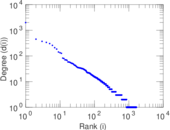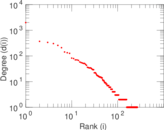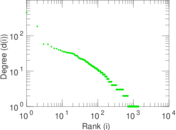### Hop distribution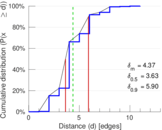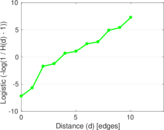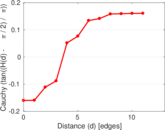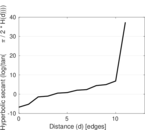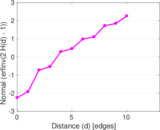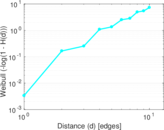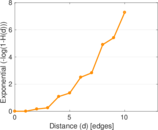### Double Laplacian graph drawing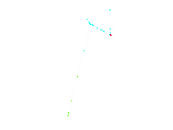### Delaunay graph drawing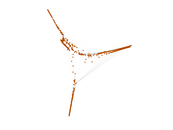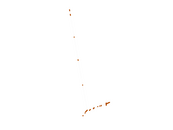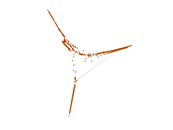### Edge weight/multiplicity distribution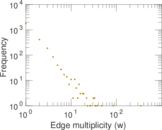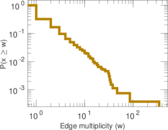### Temporal distribution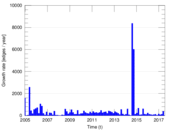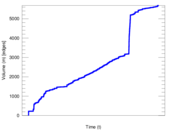### Temporal hop distribution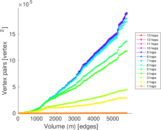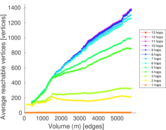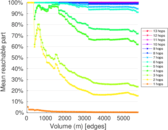### Diameter/density evolution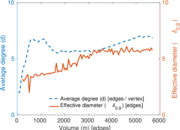### Matrix decompositions plots